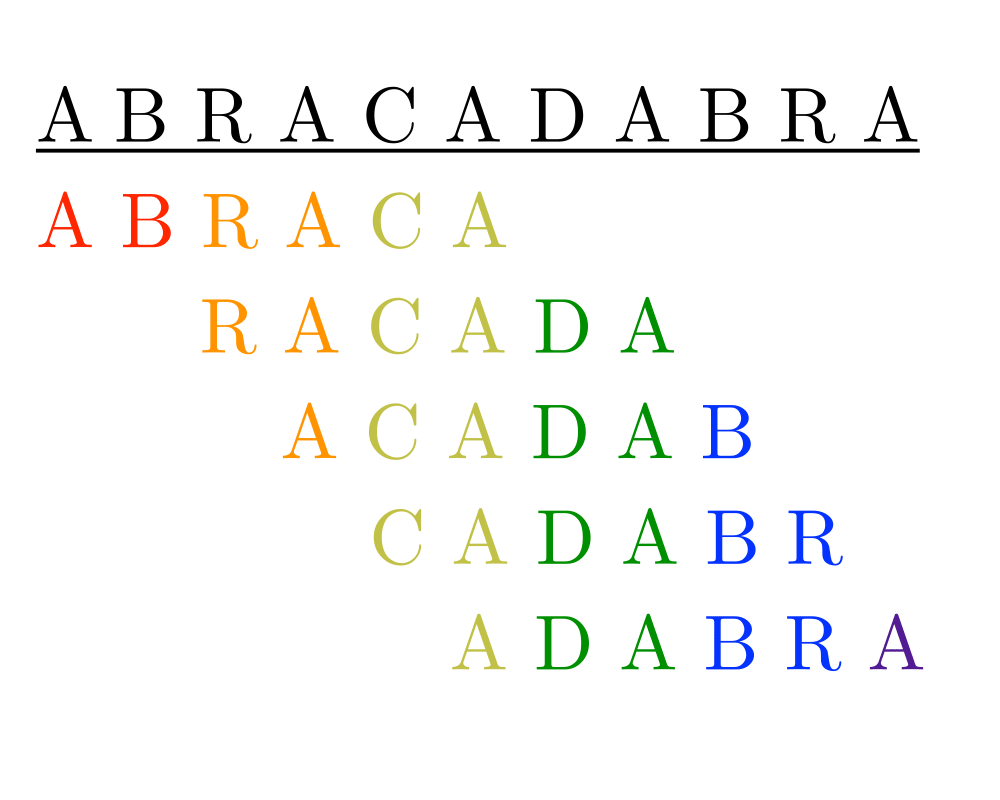# Shortest Common SuperstringInputOutput

Input Description: A set of strings $$s_1, ..., s_m$$.
Problem: Find the shortest string $$S$$ which contains each $$s_i$$ as a substring of $$S$$.

Excerpt from The Algorithm Design Manual: Shortest common superstring arises in a variety of applications, including sparse matrix compression. Suppose we have an $$n X m$$ matrix with most of the elements being zero. We can partition each row into $$m/k$$ runs of $$k$$ elements each and construct the shortest common superstring $$S'$$ of these runs. We now have reduced the problem to storing the superstring, plus an $$n X m/k$$ array of pointers into the superstring denoting where each of the runs starts. Accessing a particular element $$M[i,j]$$ still takes constant time, but there is a space savings when $$|S| << mn$$.

Go To Main Page